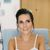# QlikView App Dev

Discussion Board for collaboration related to QlikView App Development.

Announcements
Our next Qlik Insider session will cover new key capabilities. Join us August 11th REGISTER TODAY
cancel
Showing results for
Did you mean:Creator III

## SELECT WITH ACTION

HI,

i have a button that i want that a click will make a selection in project field-

select in field

the field name -"Project"

in the search string-

='('&concat(if(Aggr((Sum({<[BUDGET VERSION]={1)}>}ActualCurrentBook_\$(vFinanceFormula)))/

(Sum({<
[BUDGET VERSION]={1}>}BudgetCurrentBook_\$(vFinanceFormula))),Project)>=1,Project),'|')&')'

and when im clicking  its diong nothing!

i put the expression in a textbox-

='('&concat(if(Aggr((Sum({<[BUDGET VERSION]={1)}>}ActualCurrentBook_\$(vFinanceFormula)))/
(Sum({<
[BUDGET VERSION]={1}>}BudgetCurrentBook_\$(vFinanceFormula))),Project)>=1,Project),'|')&')'

its gives me- (111|232|111)

what am i doing wrong?

1 Solution

Accepted SolutionsCreator III
Author

tnx good to know...

but this is not my problem...

im tring to make a selection in a field with an action.

i cant find the expression that i shoud insert

because my expression is not working and i dont know why-

='('&concat(if(Aggr((Sum({<[BUDGET VERSION]={1)}>}ActualCurrentBook_\$(vFinanceFormula)))/
(Sum({<
[BUDGET VERSION]={1}>}BudgetCurrentBook_\$(vFinanceFormula)
)),Project)>=1,Project),'|')&')'

someone?

8 RepliesCreator III
Author

?Champion III

Looks right... Try to hardcode (111|232|111), and try to use ToggleSelect, although Select must work too.

Besides, use distinct concat avoid 111 twiceCreator III
Author

hi,

tnx

i think i know what the problem bul i dont know what to do.

i will explain-

when im using the function -

GetFieldSelections(Project)

i get -  111, 222, 333 and not- (111|222|333)

i one of my other models i did get (111|222|333) and the selection worked in that model.

but i dont know why its not the same and where can i control it...

someone can help?Employee

You can define the delimitter as an argument for 'getfieldslections' and then add the '(' and ')'.

='(' &GetFieldSelections(Project , '|') & ')'Creator III
Author

tnx good to know...

but this is not my problem...

im tring to make a selection in a field with an action.

i cant find the expression that i shoud insert

because my expression is not working and i dont know why-

='('&concat(if(Aggr((Sum({<[BUDGET VERSION]={1)}>}ActualCurrentBook_\$(vFinanceFormula)))/
(Sum({<
[BUDGET VERSION]={1}>}BudgetCurrentBook_\$(vFinanceFormula)
)),Project)>=1,Project),'|')&')'

someone?Luminary Alumni

Try this expression

='('&concat(Aggr(if((Sum({<[BUDGET VERSION]={1}>}ActualCurrentBook_\$(vFinanceFormula))/

Sum({<[BUDGET VERSION]={1}>}BudgetCurrentBook_\$(vFinanceFormula)))>=1,Project), Project),'|')&')'

What is there in vFinanceFormula?  Can you elaborate what you are try to do with a sample file?

Regards,

Jagan.Creator III
Author

tnx evryone!

it was a syntax mistakeLuminary Alumni